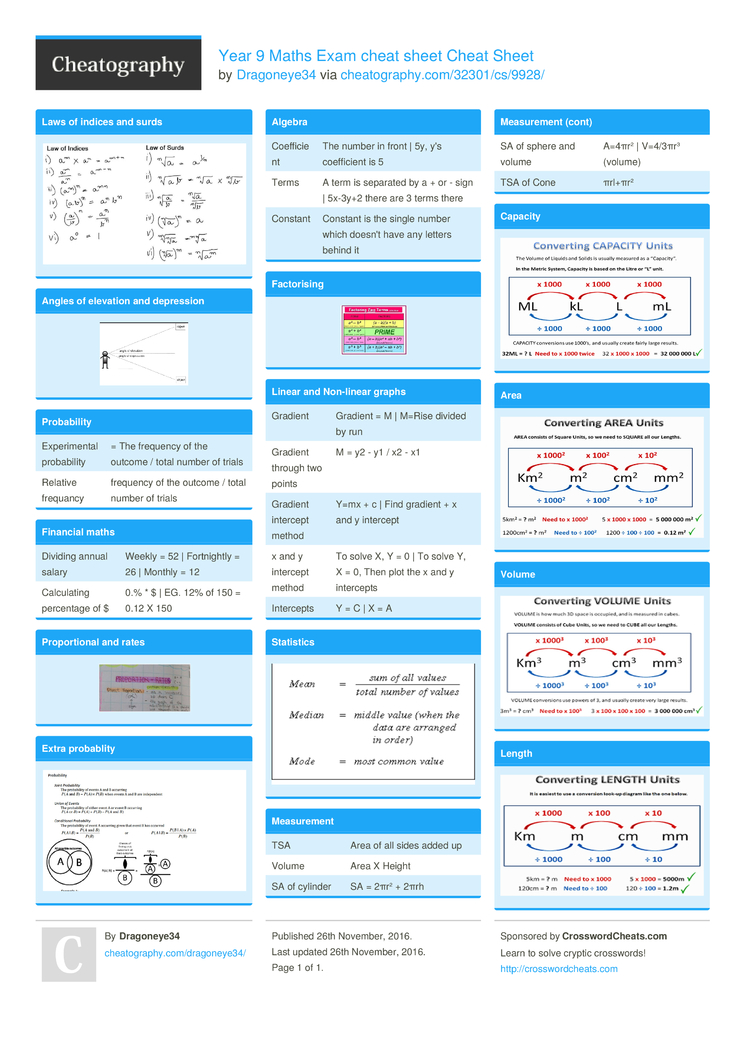# Year 9 Maths Exam cheat sheet Cheat Sheet by Dragoneye34

### Laws of indices and surds### Angles of elevation and depression### Probab­ility

 Experi­mental probab­ility = The frequency of the outcome / total number of trials Relative frequancy frequency of the outcome / total number of trials

### Financial maths

 Dividing annual salary Weekly = 52 | Fortni­ghtly = 26 | Monthly = 12 Calcul­ating percentage of \$ 0.% * \$ | EG. 12% of 150 = 0.12 X 150

### Propor­tional and rates### Extra probablity### Algebra

 Coeffi­cient The number in front | 5y, y's coeffi­cient is 5 Terms A term is separated by a + or - sign | 5x-3y+2 there are 3 terms there Constant Constant is the single number which doesn't have any letters behind it

### Factor­ising### Linear and Non-linear graphs

 Gradient Gradient = M | M=Rise divided by run Gradient through two points M = y2 - y1 / x2 - x1 Gradient intercept method Y=mx + c | Find gradient + x and y intercept x and y intercept method To solve X, Y = 0 | To solve Y, X = 0, Then plot the x and y intercepts Intercepts Y = C | X = A

### Statistics### Measur­ement

 TSA Area of all sides added up Volume Area X Height SA of cylinder SA = 2πr² + 2πrh SA of sphere and volume A=4πr² | V=4/3πr³ (volume) TSA of Cone πrl+πr²

### Capacity### Area### Volume### Length1 Page
//media.cheatography.com/storage/thumb/dragoneye34_year-9-maths-exam-cheat-sheet.750.jpg

PDF (recommended)